# 【原创】浅谈指针（十二）关于static（上）# 1.基础知识的回顾

## 1.1.内存的种类

• 全局变量
• 局部变量，也称为自动变量
• 使用malloc分配的区域
• 常量、字符串字面量

# 2.static的相关用法

## 2.2.static的地址

``````#include<iostream>
#include<windows.h>
using namespace std;
int a;//全局变量
static int b;//全局static变量
void f(void){
static int c;//定义在函数内的static变量
printf("c..%p\n",&c);
}
int main(){
printf("a..%p\n",&a);
printf("b..%p\n",&b);
f();
return 0;
}

``````

（注：今天我换了一台电脑进行编辑，使用的是codeblocks来编辑，编译器我设置的是VC）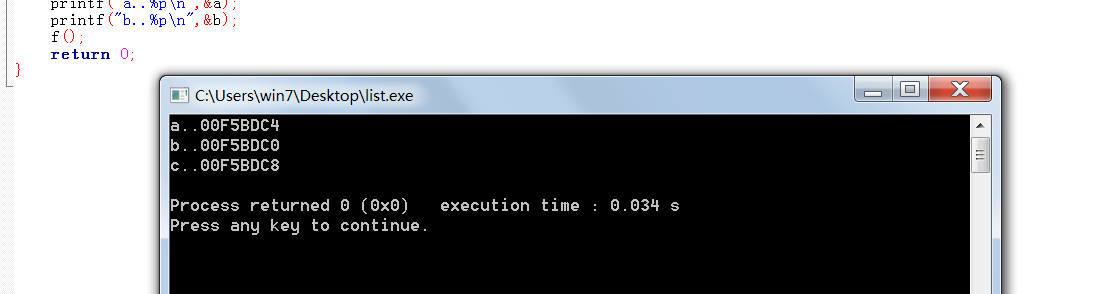## 2.3.函数体内的static

``````#include<iostream>
#include<windows.h>
using namespace std;
char *toint(int x){
char s;
sprintf(s,"%d",x);
return s;
}
int main(){
char s,t;
strcpy(s,toint(8));
strcpy(t,toint(10));
printf("%s\n%s\n",s,t);
return 0;
}

``````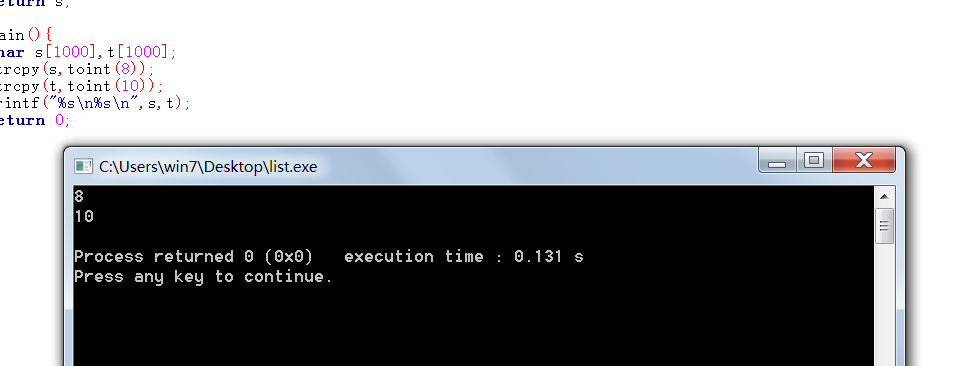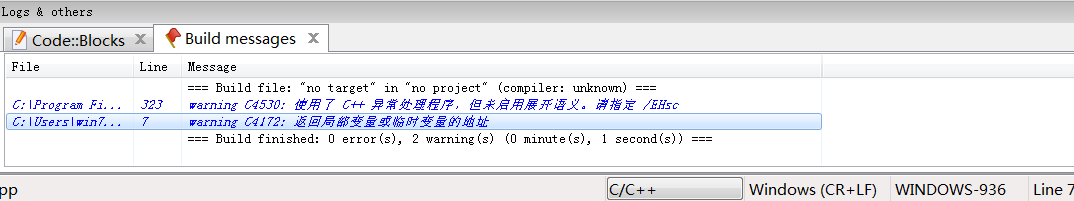（看我选中的一条，上面一条似乎是环境没有配置到位，先不管了）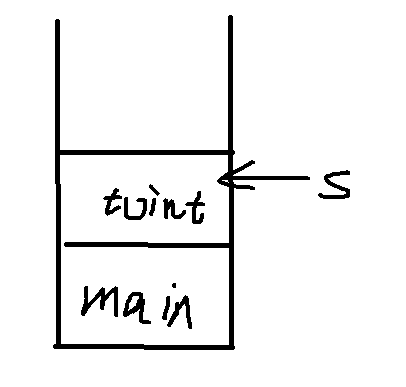``````char *toint(int x){
char *s=new char ;
sprintf(s,"%d",x);
return s;
}

``````

``````char *toint(int x){
static char s;
sprintf(s,"%d",x);
return s;
}
``````

## 3.1.在函数退出后，static变量的值保持不变

``````#include<iostream>
#include<windows.h>
using namespace std;
void f(){
static int Count;
printf("%d\n",Count);
Count++;
}
int main(){
for(int i=0;i<10;i++){
f();
}
}

``````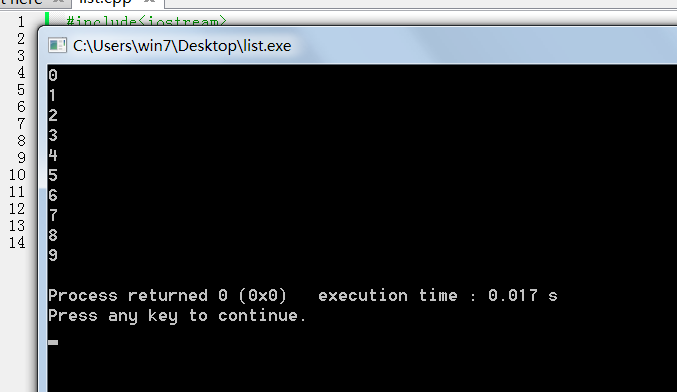## 3.2.多文件中的使用

static的变量，只能在当前的文件内进行访问。

``````//a.cpp
static int x;

int main(){
x=100;
cout<<x<<endl;
f();
}

//b.cpp
extern int x;
void f(){
cout<<x<<endl;
}
``````

``````static int f();
``````

【原创】浅谈指针（十三）关于static（下）

（预计5月发布）

2 total views,  1 views today